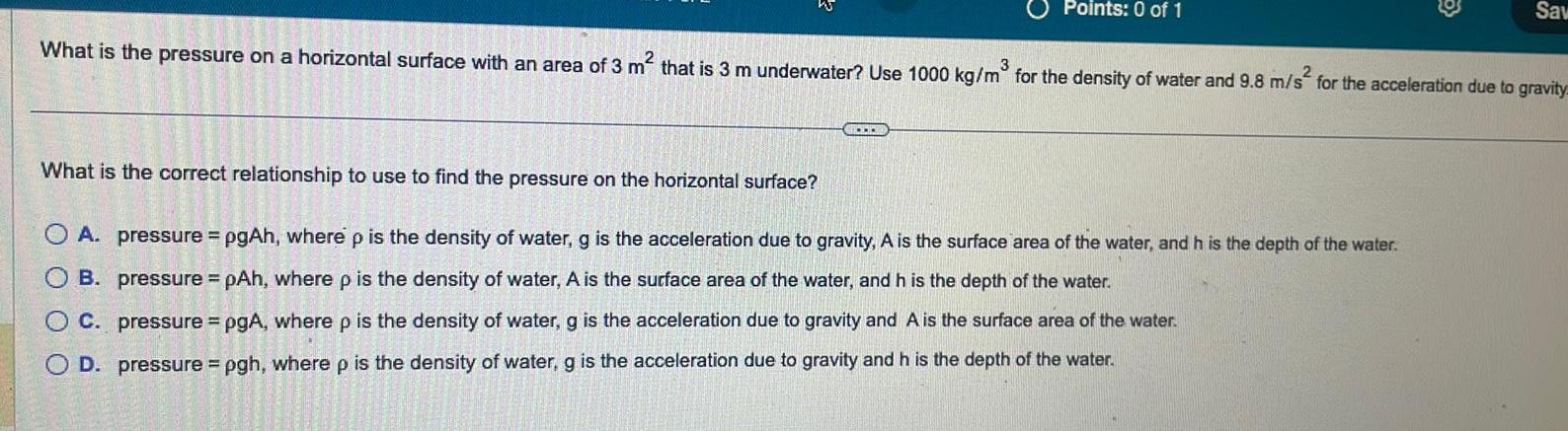Question:

# What is the pressure on a horizontal surface with an area of

Last updated: 11/19/2023What is the pressure on a horizontal surface with an area of 3 m that is 3 m underwater Use 1000 kg m for the density of water and 9 8 m s for the acceleration due to gravity OOO Points 0 of 1 30 3 1 100 What is the correct relationship to use to find the pressure on the horizontal surface OA pressure pgAh where p is the density of water g is the acceleration due to gravity A is the surface area of the water and h is the depth of the water B pressure pAh where p is the density of water A is the surface area of the water and h is the depth of the water OC pressure pgA where p is the density of water g is the acceleration due to gravity and A is the surface area of the water D pressure pgh where p is the density of water g is the acceleration due to gravity and h is the depth of the water C Sav